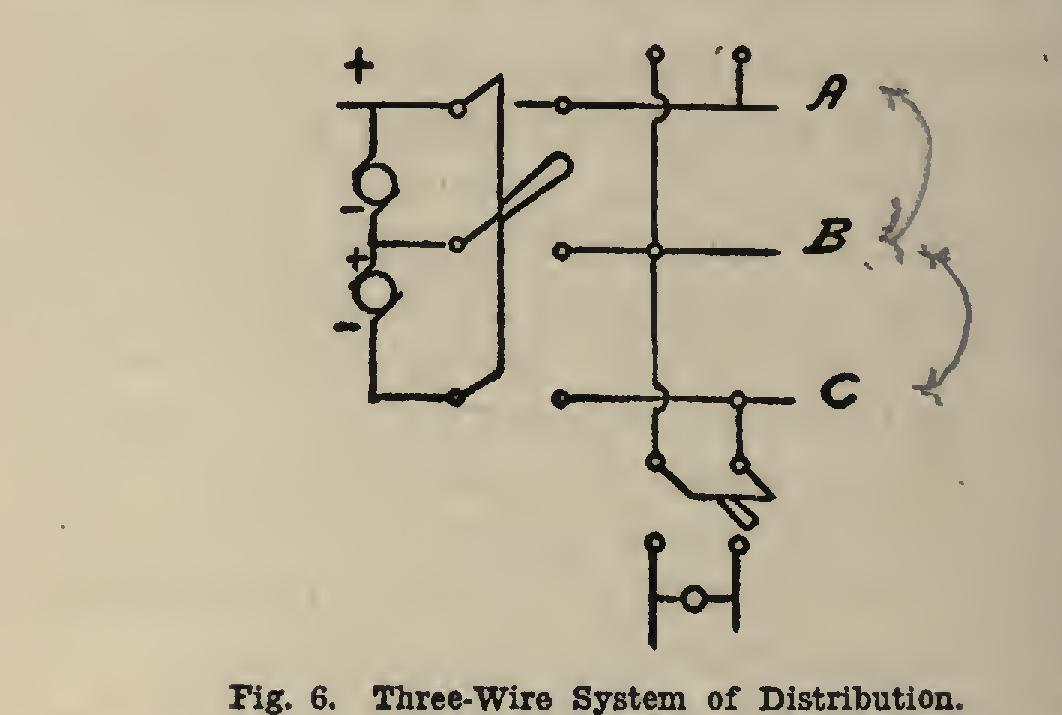Home >> Radfords-cyclopedia-of-construction-vol-4-masonry-wiring >> 325 At Load Moment to Wrought Iron 9 >> Illumination Calculations

Illumination Calculations

lamp, candle and candle-power

ILLUMINATION CALCULATIONS Incandescent lamps are usually designated as being of so many candle-power, meaning they give a certain number of times the light given by a standard candle of definite dimensions and burning at a certain rate. The efficiency of a lamp is based upon the relation of the candle power output to the watts input. The ordinary 16-candle-power carbon filament lamp requires an input of approximately 56 watts, or it has an efficiency of 3.5 watts per candle. The tungsten lamp has an efficiency of approximately 1.25 watts per candle; and the tantalum lamp, ap proximately 2.0 watts per candle. The candle power of a lamp is not the same in all directions, but depends upon the construction of the lamp.

After it is installed, candle-power in different directions will depend upon the position of the lamp, and upon the kind of reflector or shade used. In Fig. 7, the length of line from 0 to curve A in any direction, is proportional to the candle-power of lamp in that direction. Curve B gives the distribution of light when an ordi nary tin shade is used, the inner surface being enameled.Assuming that we know the general form of such curves for various lamps and reflectors, we can now calculate the candle-power required to give a certain illumination. The illumination

on any surface is measured in a unit called the foot-candle, it being the light thrown on a sur face one foot from a standard candle. Its value will vary directly as the candle-power of the source of light, and inversely as the square of the distance from same. This means that a 16-candle-power lamp will give twice as much illumination as an 8-candle-power lamp, 4 times as much as a 4-candle-power lamp, etc.; also, that if a lamp gives a certain illumination at 1 foot distance, it will give only one-fourth as much at twice the distance, one-ninth as much at 3 times the distance, one-sixteenth as much at 4 times the distance, etc.

In Fig. 7, the candle-power along the 45 degree line from curve B is 19. The illumina tion 3 feet from the lamp would be foot-candles.

The approximate values of the illumination for various parts of a residence are as follows: Knowing the required illumination, the candle-power required can be calculated.